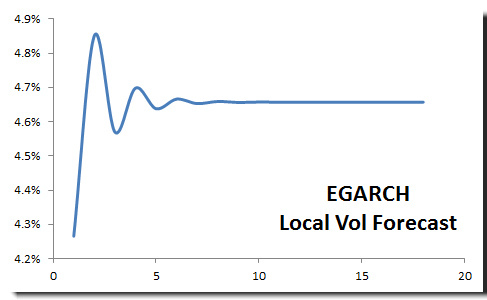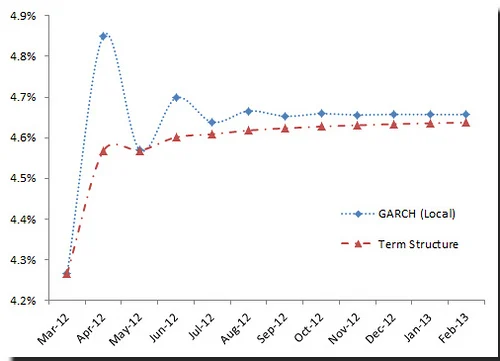# Volatility 2: Future Volatility Modeling

This is the second entry in our ongoing series of volatility modeling. In the first issue, we introduced the broad concept of volatility in financial time series, explored its general characteristics, and discussed a few non-parametric methods to estimate volatility using historical data.

### Why should you care?

The concepts discussed here are pivotal to a solid understanding of financial time series volatility. The concepts are not unique to one model, but rather generic and applicable to the entire volatility modeling domain.

In this issue, we start by defining the various terms in an asset’s return time (e.g. holding period) and explain in detail the multi-period forecast of returns and volatility. Next, we discuss the scaling issue with volatility computed with different holding periods and establish a common scaling or unit base. Finally, we define the different types of volatility terms (e.g. local volatility, term structure, long-run and forward volatility) that we will come across in our future volatility modeling.

## Background

Let’s consider the asset’s log return definition:

$$R_t=\frac{S_t}{S_{t-1}}-1$$ $$r_t=\ln{\frac{S_t}{S_{t-1}}}=\ln{S_t}-\ln{S_{t-1}}=(1-L)\ln S_t=\bigtriangledown \ln S_t$$

Where:

• $S_t$ is the closing asset’s price at end of interval t
• $S_{t-1}$ is the closing asset’s price at end of the prior interval (t-1)
• $\bigtriangledown$ is the first-order difference operator

Taking the log-returns is advantageous on three main fronts: (1) the log operator converts division operation into simple subtraction, and (2) the log transformation disperses the range over the whole real number domain $r_t \in (-\infty, \infty)$, where $R_t \in [-1,\infty)$, and finally, aggregating returns over multi-periods is a simple addition with log returns, vs. multiplication in the case of the regular returns.

$$1+R_{t\rightarrow t+k}=\frac{S_{t+k}}{S_t}=\frac{S_{t+1}}{S_t}\times\frac{S_{t+2}}{S_{t+1}}\times\cdots\times\frac{S_{t+k}}{S_{t+k-1}}=\prod_{i=1}^k \frac{S_{t+i}}{S_{t+i-1}}$$

versus

$$r_{t \to t+k}=r_{t+1}+r_{t+2}+ \cdots + r_{t+k}=\sum_{i=1}^k r_{t+i}$$

In sum, Log returns are easier to work with and they are better distributed than gross returns.

Holding period: In our definition above, we defined the log return over one period (sample step). This period is often referred to as a holding-period, and we model the dynamics of the returns with this particular holding period. The probabilistic model for returns with a given holding period can be very different from another model using a different holding period from the same asset.

Also, we can show how to compute a multi-period return forecast by simply aggregating the single-period returns:

$$E[r_{t\to t+k}]=E[r_{t+1}+r_{t+2}+\cdots + r_{t+k}]=\sum_{i=1}^k E[r_{t+i}]$$

What about a higher frequency? Can we use month returns to compute weekly? The dynamic of weekly returns is different from monthly (aggregate), so from a practical point of view we would be better off modeling weekly data, if possible, rather than monthly.

$$\textrm{Var}[r_{t\to t+k}]=\textrm{Var}[r_{t+1}+r_{t+2}+\cdots + r_{t+k}]=\sum_{i=1}^k \textrm{Var}[r_{t+i}]+2\times\sum_{i=1}^{k-1}\sum_{j=i+1}^k\textrm{Cov}[r_{t+i},r_{t+j}]$$

Where:

• $\textrm{Cov}[r_{t+i},r_{t+j}]$ is the covariance between $r_{t+i}$ and $r_{t+i}$

In the asset’s returns time series, it is not uncommon to find the returns to be serially uncorrelated, yet they are dependent (e.g. volatility clustering). This outlines that the inter-relationship is higher-order than linear correlation.

Assuming we have a weak-sense stationary (WSS) process and returns are not serially correlated, then multi-period variance (i.e. squared-volatility) is expressed as follows:

$$\textrm{Var}[r_{t\to t+k}]=\sum_{i=1}^k \textrm{Var}[r_{t+i}]$$

## Volatility Scaling

To compare the value of single-period volatility against multi-period volatility, we need a unified scale or unit-base. In practice, annual volatility is often implied when discussing values. The question now becomes, how do we convert a monthly, weekly, or daily volatility to annual?

For monthly volatility, we compute 12-month period volatility assuming no serial correlation between returns:

$$\sigma_Y^2=12\times\sigma_{mo}^2$$ $$\sigma_Y=\sqrt{12}\times\sigma_{mo}$$

For weekly volatility, we compute 52 week period volatility assuming no serial correlation between individual weekly returns:

$$\sigma_Y=\sqrt{52}\times\sigma_{w}$$

For daily volatility, the holding period is a trading day, so first we compute the number of trading days in the current year (in the US, $52\times 5 - 5=250$ trading days), and then multiply by the square root of this number:

$$\sigma_Y=\sqrt{250}\times\sigma_{d}$$

## Local, term structure, forward and long-run Volatility

In time series, we often mention conditional, marginal, or unconditional and long-run volatility. For many of us, it is easy to get them confused, so let’s spell out their definitions now.

Local Volatility

Local volatility is an acronym for one-step conditional volatility but is often used with volatility forecasts.

$$\sigma_{t\to t+1}=f(t,r_t,r_{t-1},\cdots,r_1)$$

For instance, the moving window standard deviation defines next step volatility in terms of the last m returns.

$$\sigma_{t\to t+1}=\sqrt{\frac{\sum_{i=1}^m (r_{t-i}-\bar r)^2}{m-1}}$$

In the Volatility forecast NumXL Tips & Hints issue, we constructed an EGARCH model to forecast monthly local volatility of S&P 500. The graph is shown below:Long-run volatility

One of the important characteristics of volatility in financial time series is the reversion-to-the mean property. The volatility might go high for a period of time, then surely come down to its historical level, or it can be low over an extended period of time to go higher to a long-run level.

In notation

$$\sigma_{LR}=\lim_{k\to \infty}\sigma_{t+k}$$

Long-run volatility is what is perceived as the historical level; this is not the same as the sample standard deviation, and it does vary based on the model that captures its dynamics.

Again, in the volatility forecast issue, the local volatility converges to fixed values as the number of forecast steps >>1 (~4.66%). Please, note the values are expressed in a monthly unit, not annual. To compute the annual long-run volatility;

$$\sigma_A=4.66\% \times \sqrt{12}=16.14\%$$

The S&P 500 has historical volatility of around 16% per Annum.

Nevertheless, there are non-parametric methods (smoothing filters) to estimate long-run volatility, for instance, a Bartlett kernel filter with window size k (refer to the NumXL LRVar function).

Term Structure

The term structure is basically a function that defines the volatility’s values for different future holding periods.

Using today ($T$) as reference date, the volatility term structure is defined as follows:

$$\sigma_{t\to t+k}^2=f(t,k|F_{t})=\frac{\sigma_{t\to t+1}^2+\sigma_{t+1\to t+2}^2+\cdots+\sigma_{t+k-1\to t+k}^2}{k}$$

Note that we divided the sum of local variances by the number of periods to preserve the scaling of the volatility (i.e. annual variance).

Using the EGARCH volatility forecast for S&P500 in an earlier issue (March 12, 2012 NumXL Tips & Hints Issue – Volatility Forecast), we plotted the local volatility forecast along with term structure.Again, the volatility values are for monthly volatility (i.e. not scaled to annual).

Forward Volatility

In the term structure definition, all volatility values are for a holding period from the reference date (today) and some future date. What if we wish to estimate volatility between two future dates? Well, this is what forward volatility is about:

$$\sigma_{t\to t+k}^2=\frac{\sigma_{t\to t+1}^2+\sigma_{t+1\to t+2}^2+\cdots+\sigma_{t+k-1\to t+k}^2}{k}$$ $$\sigma_{t\to t+k}^2=\frac{1}{k}((\sigma_{t\to t+1}^2+\sigma_{t+1\to t+2}^2+\cdots+\sigma_{t+m-1\to t+m}^2) + (\sigma_{t+m\to t+m+1}^2+\sigma_{t+m+1\to t+m+2}^2+\cdots+ \sigma_{t+k-1\to t+k}^2))$$ $$\sigma_{t\to t+k}^2=\frac{1}{k}(m\times\frac{\sigma_{t\to t+1}^2+\cdots+\sigma_{t+m-1\to t+m}^2}{m} + (k-m)\times\frac{\sigma_{t+m\to t+m+1}^2+\cdots+ \sigma_{t+k-1\to t+k}^2}{k-m})$$ $$\sigma_{t\to t+k}^2=\frac{m\times\sigma_{t\to t+m}^2+(k-m)\times\sigma_{t+m\to t+k}^2}{k}$$

Thus;

$$\sigma_{t+m\to t+k}^2 = \frac{k\times\sigma_{t\to t+k}-m\times\sigma_{t\to t+m}^2}{k-m}$$

Note:

The local (conditional) volatility is basically a one-step forward volatility.

## Correlated Returns & Time-Varying Volatility

Let’s assume we have a time series with correlated returns. How do we compute multi-period volatility? How do we proceed?

Well, we decompose the asset's returns into two components:

$$r_t=\mu_t+a_t$$ $$r_t-\mu_t=a_t$$

Where:

• $\mu_t$ is the conditional mean (non-stochastic)
• $a_t$ is the stochastic shock or error term
• $E[a_t]=0$
• $\mathrm{Var}[a_t]=\mathrm{E}[a_t^2]=\sigma_t^2$

Thus,

$$\mathrm{Var}[r_t]=\mathrm{Var}[a_t]=\sigma_t^2$$

Furthermore, the data points of the $\{a_t\}$ time series are not assumed to be either independent or identically distributed. Instead, $\{a_t\}$ observations need only to be serially (i.e. first-order) uncorrelated.

In short, everything we have done earlier is still applicable, but using the mean-corrected (i.e. residuals) time series $\{a_t\}$.

Hmm.. We don’t know the conditional mean? In that case, things can get a bit more complicated; we need to use two models: conditional mean and conditional volatility models.

We will leave this topic for a future issue.

## Conclusion

In this issue, we did not assume any model for the asset’s returns time series and proceeded to lay the groundwork for volatility modeling.

Furthermore, we showed that the absence of serial correlation in the series returns is sufficient to simplify the multi-period volatility calculation. That leaves us with just one question: what if we have a serial correlation?

Finally, we discussed the possibility of a process with serially correlated returns and volatility that varies over time (first and second-order dependency). We are leaving this type of modeling to future issues.